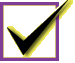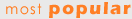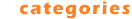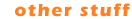Category: Education : MathematicsFileProfile Quick Pick: Acrony Our Most Popular Download and the Editor's Top Pick is a multi-player word game named Acrony. It's easy to install, quick to learn and extremely addictive. Try it!Rank Title - Education : Mathematics DownloadsCalc 3D (\$10) - Free calculator for vectors, matrices, complex numbers and quaternions 2048 2 Math Homework Maker (\$0) - Math Homework Maker,is a FREE software which can solve all your math homework 2031 3 MultyGraphiX (\$29.90) - MultyGraphix is the mathematical software designed to plot 2D and 3D functions 1994 4 GEUP (\$35) - Discover the power of Interactive Geometry for learning and doing Mathematics 1930 5 Linear Systems Beams (\$0) - Macintosh freeware for middle school mathmatics 1928 6 Graphing Functions (\$0) - Macintosh freeware for middle school mathmatics 1925 7 Math Flight (\$11.95) - Fly High while having fun learning basic mathematics with Math Flight! 1925 8 MatrixCalculator (\$10) - Simple pocket calculator style matrix calculator 1918 9 Random Test Generator-PRO (\$99.95) - Create Tests from Randomly Selected Questions of test item databanks 1913 10 MathAid Algebra II (\$31.95) - Contain basic concepts, interactive examples, problems and customizable tests 190111 Triangle Types (\$0) - Macintosh freeware for middle school mathmatics1898 12 License Plate Math (\$14.99) - A fun math puzzle game you can also play while driving. 1877 13 MathAid Trigonometry (\$27.95) - Java based math course includes problem-solving lessons and tests 1826 14 MadCalc (\$10) - MadCalc is a full featured graphing calculator application for Windows. 1741 15 Integer Balance Beam Puzzles (\$0) - Macintosh freeware for middle school mathmatics 1739 16 Angle Doubling (\$0) - Macintosh freeware for middle school mathmatics 1737 17 MB-Ruler (\$0) - tool to measure distances and angles on the desktop + screen loupe 1708 18 CalcBoard (\$14.95) - Calculate expressions using units and interval arithmetic 1699 19 SplineCalc (\$75) - Multipurpose scientific calculator with ample opportunities and high precision 1670 20 Chase (\$0) - Macintosh freeware for middle school mathmatics 165221 Multi Maze Mountain 2 (\$10) - Multi Maze Mountain is a mazegame dealing with the multiplication tables.1647 22 Rt-Plot (\$50.00) - Rt-Plot is a tool to generate Cartesian X/Y-plots from scientific data. 1636 23 KwikTrig (\$15.00) - For quick solutions to trigonometry and geometry problems from expert to novice. 1582 24 Math Worm (\$10.00) - A math puzzle where your worm navigates a maze completing arithmetic equations. 1556 25 pmaCalc (\$0) - pmaCalc is the replacement for your pocket calculator 1525 26 Evaluating Crossnumber Puzzles (\$0) - Macintosh freeware for middle school mathmatics 1518 27 CFB Primes (\$0) - Find the prime factors and closest primes of any integer up to one billion (US) 1458 28 Mosquito (\$0) - Macintosh freeware for middle school mathmatics 1334 29 Graphit (\$5) - Low cost 2D function plotting software. Function comparison, delta position 1320 30 Snowflakes & Stars (\$0) - Draw colorful snowflakes and stars having from 4 to 90 sides in a few clicks! 125931 LeoStatistic (\$89.95) - Universal statistical analysis and modeling of experimental and market data.1258 32 Sebran's ABC (\$0) - Learn letters and numbers 1186 33 MathMatic Junior Edition (\$0.00) - Multiplication tables driller 1 through 12. Learn your times tables with fun. 1179 34 mWorksheet (\$20) - Create math worksheets for addition, subtraction, multiplication & division. 1149 35 Abacus Math Writer (\$49.00) - For publishing Mathematical and Scientific equations. 1125 36 Fraction Puzzles (\$0) - Macintosh freeware for middle school mathmatics 1113 37 Algebra - One On One (\$14.99) - Makes learning algebra fun. 21 skill levels. Learn step by step. 1097 38 GraphiCal (\$20) - Programmable calculator creates graphs & video clips from expressions & formulas 1083 39 FNGraph (\$0) - Graphing and analyzing mathematical functions 1068 40 Micro Economy Model (\$29.90) - For studying: Price-Demand, Income, Cost and Benefit, behavior. 106641 DeadLine (\$0) - Solve equations graphically and numerically.961 42 MaterialWorlds Simulations (\$19.95) - Play and experiment with virtual reality simulations. 961 43 Arcs & Sectors (\$0) - Macintosh freeware for middle school mathmatics 943 44 mBasics (\$35) - Create math worksheets & assign to students for testing. 935 45 Number Line (\$0) - Macintosh freeware for middle school mathmatics 919 46 Mocha (\$0) - Sound reactive visualizations of mathematical equations. 866 47 MathAid College Algebra (\$30.95) - Java based math course includes problem-solving lessons and tests 834 48 Graphmatica (\$25) - powerful, easy-to-use, equation plotter with numerical and calculus features 826 49 Linear Algebra (\$15) - Performs computations associated with matrices. 789 50 Calc 3D Pro (\$0.00) - Calc 3D is a collection of mathematical tools for highschool and university. 77351 DigitizeIt (\$39) - DigitizeIt can automatically digitize scanned graphs and output data values.762 52 Math Function Mania (\$14.99) - Learn algebraic functions with this multi-level math game. 748 53 3D Grapher (\$24.95) - Plot and animate 2D and 3D equation and table-based graphs with ease 734 54 LangPad - Math & Currency Characters (\$7) - Insert Math & Currency characters & symbols into WordPad & Notepad text 733 55 SLAE Solver (\$10.95) - SLAE SOLVER allows to find on a personal computer high accuracy solutions 726 56 Weights And Measures Plus (\$24.95) - Unit conversions, Fractions, Trigonometry, Roman Nums, World Currency Exchange 718 57 Brain Builder - Math Edition (\$14.99) - Over 500 million math puzzles in a multimedia game. Has 16 skill levels. 682 58 CaterpillarSSA (\$99.5) - The program for extended Singular Spectrum Analysis (SSA) of time series. 664 59 Animated Arithmetic (\$19.00) - Teaches addition, subtraction, multiplication and division for 1st - 4th grades. 657 60 Magic Graph (\$19.95) - Plot and analyze graphs of mathematical functions with this powerful tool. 62261 Box & Whiskers Drill (\$0) - Macintosh freeware for middle school mathmatics610 62 Data Master 2000 (\$0) - Automation of measurement systems, data acquisition, processing, analysis 601 63 fplot@t-st.de (\$0) - Plots functions in the form y=f(x) or in the implicit form f(x,y)=0 554 64 Pizza Party Puzzles (\$0) - Macintosh freeware for middle school mathmatics 543 65 Basic Algebra Shape-Up (\$35.00) - Hands-on algebra tutorials covering integers, expressions, equations, and more. 524 66 CalcCoord (\$11) - transforms cartesian, spherical and cylindrical coordinates 487 67 Adding Three Fractions (\$0) - Macintosh freeware for middle school mathmatics 486 68 statistiXL (\$75) - A powerful statistics and statistical analysis add-in for Microsoft Excel 480 69 MathAid Precalculus (\$32.95) - Contain basic concepts, interactive examples, problems and customizable tests 465 70 CalculPro (\$20.00) - CalculPro to practise mental arithmeticâ��s or fractions. 45571 Fraction Tiles (\$0) - Macintosh freeware for middle school mathmatics453 72 HiDigit (\$19.95) - Powerful calculator with extended capabilities for students and teachers. 432 73 \$1.64 Puzzle (\$0) - Macintosh freeware for middle school mathmatics 400 74 GraphSight Junior (\$0) - GraphSight Junior is an easy to use freeware handy 2D math-graphing program. 330 75 20sim Viewer (\$0) - An advanced modeling and simulation program for dynamic systems. 306 76 SimplexNumerica (\$200.00) - Best data analyzer, 2D/3D-plotting, calc and presentation program. 278 77 Flashcard Tables (\$7.95) - Practice simple mathematical operations for speed. 225 78 Flash Math (\$2.00) - Flash Math duplicates math flash cards 221 79 Graph (\$0) - Program for drawing graphs of mathematical functions in a coordinate system. 177 80 tApCalc Scientific tape calculator(Arm) (\$7.95) - Programmable scientific tape calculator that allows save,edit,run tape. 16381 GraphSight (\$29) - Feature-rich comprehensive 2D math graphing utility with easy navigation.160 82 Animated Math (\$15.00) - Teaches counting, addition and subtraction with animated rewards. 143 83 Rewards Multiply (\$19) - A rewarding game that motivates children to learn their multiplication tables. 126 84 Calcul (\$0.00) - CALCUL to practise mental arithmeticâ��s or fractions. 100 85 Percent-O-Rama (\$0) - Macintosh freeware for middle school mathmatics 95 86 Slot Machine Clinic (\$0) - Macintosh freeware for middle school mathmatics 80 87 Image++ (\$49) - The Object-oriented graphic development tool for digital image processing and Ma 21 88 Karnaugh Minimizer (\$20) - Boolean Algebra assistant program 2 89 Prime Number Spiral (\$16.00) - Software for exploring the Prime Number Spiral (a.k.a. the Ulam Spiral) 1 90 Multiplication Table (\$6) - To learn the multiplication table!? There is nothing easier! 191 ESBPDF Analysis - Probability Software (\$29) - Probability Analysis Software for Windows that is easy to use.1 92 3D Geometrical Objects (\$29) - Represents prisms and pyramids in 3D space. Supports interactive 3D animation. 0 93 MathCards (\$10.00) - MathCards helps students reinforce their math skills by building math equations. 0Most Popular Editor's Choice Category Listing Education -Computer -Dictionaries -Geography -Kids -Languages -Mathematics -Other -Reference Tools -Science -TeachingSubmit Software Contact Us Terms of Use Other Sites Advertising

 Copyright 2004 by FileProfile.com